SELF 8 S.B. Karavashkin and O.N. KaravashkinaThus, having begun with quite doubtful supposition as to the theory which Einstein does not conceive, but in which for certain has to be true a convenient for him condition of zero tensor of energy, he derives a thesis of doubt, whether he can under this condition yield the weighty matter - and finishes in persuasion that (44) is true always. Hence, there is also true the condition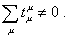(45)
 Immediately arise few questions, and first of all, where from the conditions (43) and (44) appeared? “In the initial theory of relativity the conservation law of pulse and energy is expressed by the equation of the kind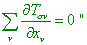(46)
 [18, p. 323]. It is clear that (44) appeared from the condition of conservative system. But with it the very tensor of energy-pulse does not determine exceptionally the electromagnetic either gravity field. “The energetic properties of the system are characterised by the energy tensor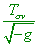(47)
 (mixed tensor). As the energy relates to inertia and inertia - to the weight, gravity field has to be determined by the values T” [18, p. 321]. Since SR, as is known, did not account the gravity interaction but considered only electromagnetic field, the energy-pulse tensor has to determine both electromagnetic and gravity field. But in passing from SR to GR, there appear difficulties with the energy-pulse conservation law, because of which in the end of ends Einstein denied conservation laws and prooflessly introduced his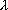-term. The difficulties arose from the very beginning. “The corresponding equation yielded with the absolute differential calculus says that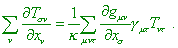(48)
 The equation (48) already does not express the conservation law. From the physical view this is understandable, as the substance taken separately cannot satisfy the conservation laws in presence of gravity field, because the gravity field passes the pulse and energy to the substance. The right part of (48) expresses it. However, if conservation laws have to exist at all, it is necessary to require, these laws in the form (46) to be true for the substance and gravity field together. Then the system of equations like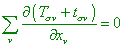(49)
 has to exist, where the values tcan depend only on g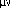and their derivatives” [18, p. 321]. It is understandable that from (49) there follows the condition (43) by which Einstein substituted the conservation law (44). But firstly, such substitution is not so much obvious even from the view of conception he developed, as “however, generally covariant systems of equations like (49) do not exist” [18, p. 323]. Well, if so, what is the sense to substitute this, which, by Einstein’s opinion, is invalid in general case by that which does not exist? Secondly, as we revealed before, in general case the energy-pulse tensor determines not only gravity but also electromagnetic interaction. With it, if we follow Einstein, in gravitation field the conservation law has to be recorded as (49), as the gravity field changes the energy of studied body. At the same time, with the electric interaction which is an indivisible part of electromagnetic interaction, we may use the conservation laws as (46). But if an electric interaction also was able to change the body’s energy, the initial Einstein’s admission “assume for a while that in an improved so electrodynamics, the scalar of energy tensor also turns into zero!” is wrong and we have, both in general case and in case of EM interaction, to write the conservation law as (43), with all consequences for SR. And for GR too, as equations like (43) do not exist in limits of general covariance. “Deeper study shows that such systems are covariant only in relation to linear transformations. Consequently, requiring to formulate the equations of gravity field so that to provide the conservation laws to be true, we limit our choice of the reference frames so that only linear transformations can transfer one allowed frame into another” [18, p. 323]. Namely this condition caused Schwarzschild to confine his problem to the sought of just linear element - and we showed in our work that he factually has not fulfilled this task. At the same time, we clearly see a dual, insincere Einstein’s attitude in formulation of both SR and GR. From the full amount of physical properties, he selected some amount of revelations that were convenient for him, which, dependently on his own convenience, can be revealed either absent. Thereupon, the condition (39) appears to be the atavism of SR in GR which Einstein intentionally retained to provide the invariance of 4-metric in passing to GR. With it, Einstein himself did not care that such condition puts both mathematical formalism and phenomenology of GR onto two diverging ice floes. It was more important for him that out of this condition, GR basically could not be formulated. From this view, there become quite sensible multiple notices by Einstein like this: “if we, doing not knowing the generally covariant equations of gravity field, specialise the reference frame and compose the equations of gravity field only for this specialised frame, the theory cannot rise any objections except the only, namely - that the composed equations, possibly, have a lack of physical meaning. But in the considered case, no one will support this objection seriously” [18, p. 322]. Is it worthy after that to be surprised that writing the element of volume in spherical coordinates (38), Schwarzschild accounted what was convenient for him, omitting the coefficients G and H. But if we consider this passing in rigorous accordance with mathematical formalism, we will see that Schwarzschild, with help of his artificial trick, has not provided the Jacobian equal to unity in passing from the initial rectangular coordinates to the artificial x-coordinates, since, as we pointed in our paper, he did not account G and H. And by the way, Einstein’s (39), with account of his own determinant (20), is invalid in static gravity field. But if we consider (39) from the view of keeping the local invariant of the light speed, then with accuracy to the coefficient which tends to unity with the motion away from the field source (of course, if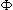tends with it to the unity, which is quite doubtful), not equal to unity in passing from one coordinate system to another, the logic of this condition remains in limits of GR. The Jacobian with it, as Einstein defined, has to determine the passing between the systems of spatial coordinates. So the claim of colleagues who tried to find our mistake, as if we did not account in our Jacobian in (21) of our work, page 6, the time coefficient F, is groundless. Because, as we already said, after the above Einstein’s opinion, the symmetry of metric has to be conserved only with respect to the spatial coordinates. Just so Schwarzschild also considered the Jacobian in 3 D form.

Contents / 1 / 2 / 3 / 4 / 5 / 6 / 7 / 8 / 9 / 10 / Paper